# Selina Solutions Concise Mathematics Class 6 Chapter 22 Simple (Linear) Equations Exercise 22(C)

Selina Solutions Concise Mathematics Class 6 Chapter 22 Simple (Linear) Equations Exercise 22(C) are curated with the intention of helping students perform better in the final exam. The problems talked about under this exercise are based on determining the value of variables. Practising solutions on a regular basis helps students to improve problem solving and analytical skills. Students who intend to obtain conceptual knowledge, can use Selina Solutions Concise Mathematics Class 6 Chapter 22 Simple (Linear) Equations Exercise 22(C) PDF, free from the links provided below.

## Selina Solutions Concise Mathematics Class 6 Chapter 22 Simple (Linear) Equations Exercise 22(C) Download PDF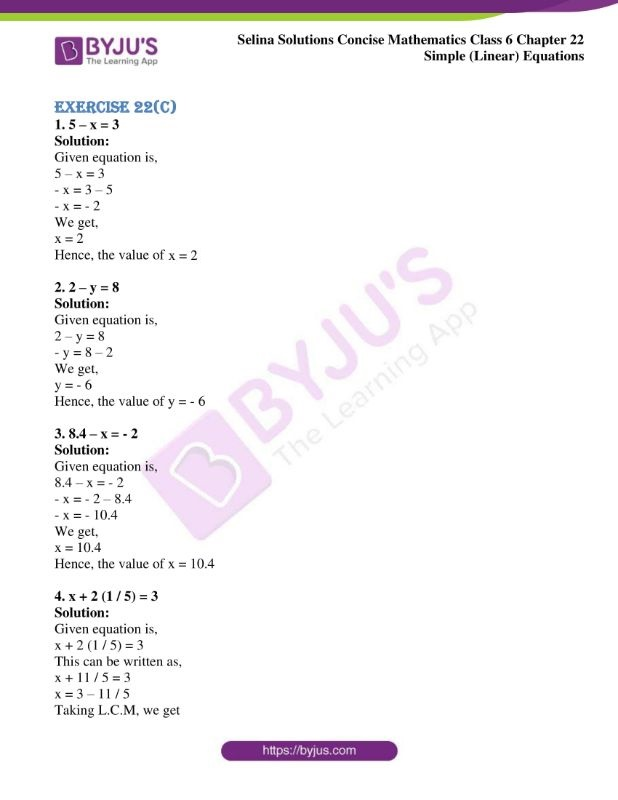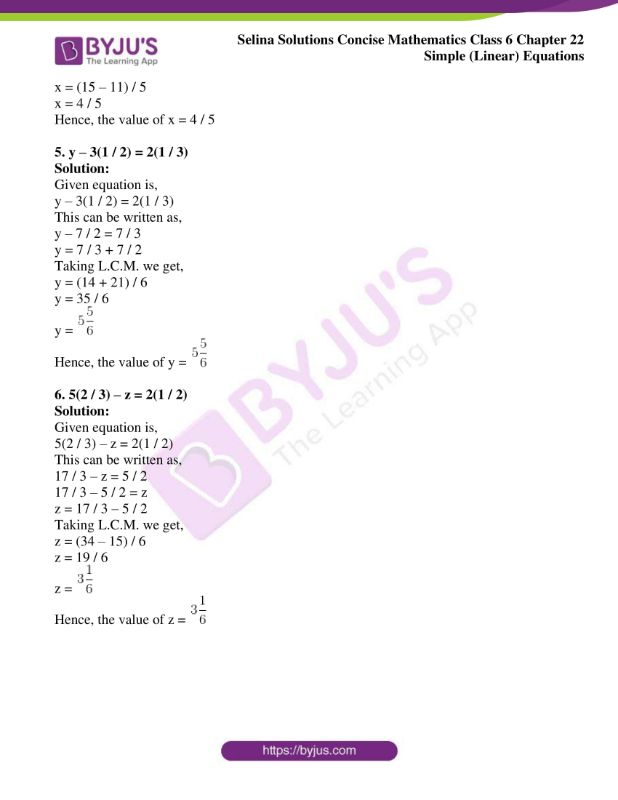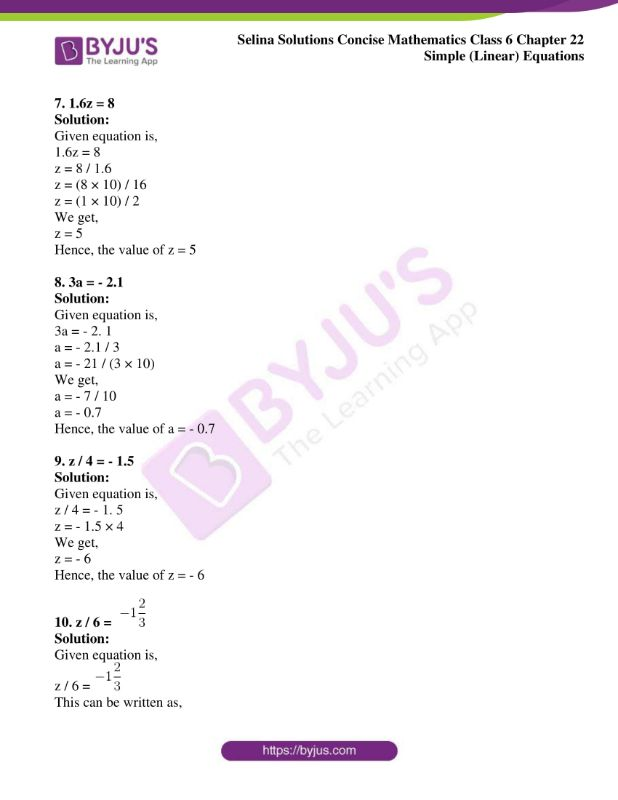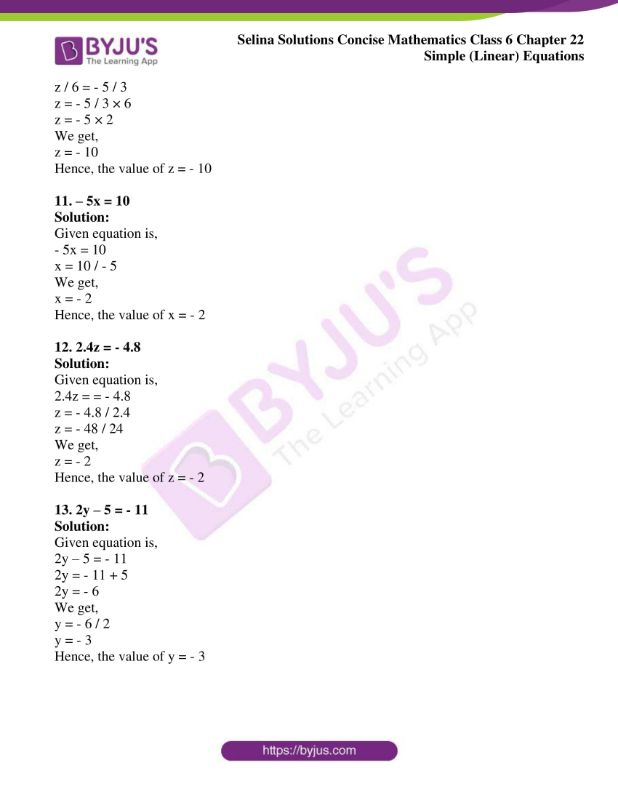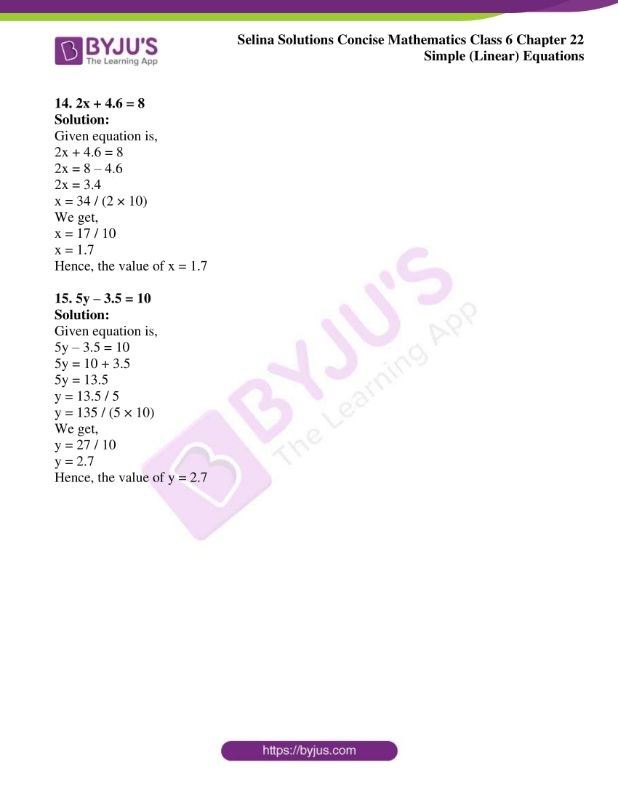### Access other exercises of Selina Solutions Concise Mathematics Class 6 Chapter 22 Simple (Linear) Equations

Exercise 22(A) Solutions

Exercise 22(B) Solutions

Exercise 22(D) Solutions

### Access Selina Solutions Concise Mathematics Class 6 Chapter 22: Simple (Linear) Equations Exercise 22(C)

Exercise 22(C)

1. 5 – x = 3

Solution:

Given equation is,

5 – x = 3

– x = 3 – 5

– x = – 2

We get,

x = 2

Hence, the value of x = 2

2. 2 – y = 8

Solution:

Given equation is,

2 – y = 8

– y = 8 – 2

We get,

y = – 6

Hence, the value of y = – 6

3. 8.4 – x = – 2

Solution:

Given equation is,

8.4 – x = – 2

– x = – 2 – 8.4

– x = – 10.4

We get,

x = 10.4

Hence, the value of x = 10.4

4. x + 2 (1 / 5) = 3

Solution:

Given equation is,

x + 2 (1 / 5) = 3

This can be written as,

x + 11 / 5 = 3

x = 3 – 11 / 5

Taking L.C.M, we get

x = (15 – 11) / 5

x = 4 / 5

Hence, the value of x = 4 / 5

5. y – 3(1 / 2) = 2(1 / 3)

Solution:

Given equation is,

y – 3(1 / 2) = 2(1 / 3)

This can be written as,

y – 7 / 2 = 7 / 3

y = 7 / 3 + 7 / 2

Taking L.C.M. we get,

y = (14 + 21) / 6

y = 35 / 6

y =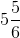Hence, the value of y =6. 5(2 / 3) – z = 2(1 / 2)

Solution:

Given equation is,

5(2 / 3) – z = 2(1 / 2)

This can be written as,

17 / 3 – z = 5 / 2

17 / 3 – 5 / 2 = z

z = 17 / 3 – 5 / 2

Taking L.C.M. we get,

z = (34 – 15) / 6

z = 19 / 6

z =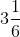Hence, the value of z =7. 1.6z = 8

Solution:

Given equation is,

1.6z = 8

z = 8 / 1.6

z = (8 × 10) / 16

z = (1 × 10) / 2

We get,

z = 5

Hence, the value of z = 5

8. 3a = – 2.1

Solution:

Given equation is,

3a = – 2. 1

a = – 2.1 / 3

a = – 21 / (3 × 10)

We get,

a = – 7 / 10

a = – 0.7

Hence, the value of a = – 0.7

9. z / 4 = – 1.5

Solution:

Given equation is,

z / 4 = – 1. 5

z = – 1.5 × 4

We get,

z = – 6

Hence, the value of z = – 6

10. z / 6 =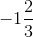Solution:

Given equation is,

z / 6 =This can be written as,

z / 6 = – 5 / 3

z = – 5 / 3 × 6

z = – 5 × 2

We get,

z = – 10

Hence, the value of z = – 10

11. – 5x = 10

Solution:

Given equation is,

– 5x = 10

x = 10 / – 5

We get,

x = – 2

Hence, the value of x = – 2

12. 2.4z = – 4.8

Solution:

Given equation is,

2.4z = = – 4.8

z = – 4.8 / 2.4

z = – 48 / 24

We get,

z = – 2

Hence, the value of z = – 2

13. 2y – 5 = – 11

Solution:

Given equation is,

2y – 5 = – 11

2y = – 11 + 5

2y = – 6

We get,

y = – 6 / 2

y = – 3

Hence, the value of y = – 3

14. 2x + 4.6 = 8

Solution:

Given equation is,

2x + 4.6 = 8

2x = 8 – 4.6

2x = 3.4

x = 34 / (2 × 10)

We get,

x = 17 / 10

x = 1.7

Hence, the value of x = 1.7

15. 5y – 3.5 = 10

Solution:

Given equation is,

5y – 3.5 = 10

5y = 10 + 3.5

5y = 13.5

y = 13.5 / 5

y = 135 / (5 × 10)

We get,

y = 27 / 10

y = 2.7

Hence, the value of y = 2.7